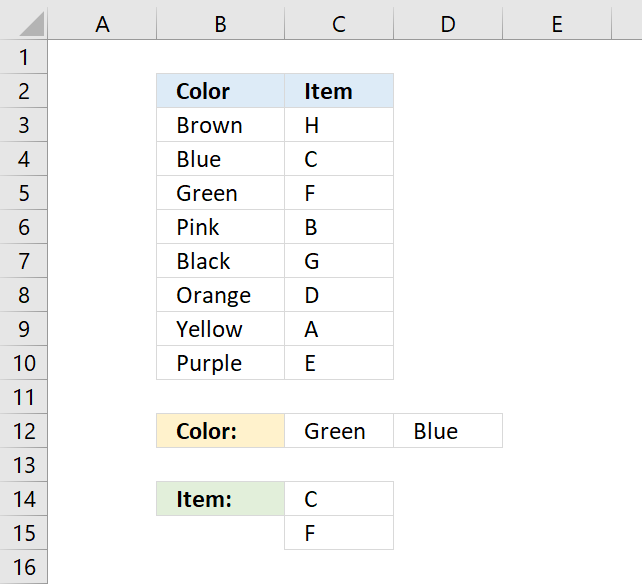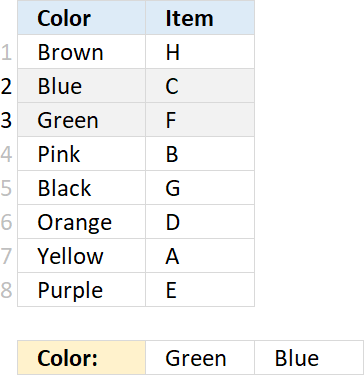Author: Oscar Cronquist Article last updated on November 15, 2018The formula in cell C14 returns multiple values from column Item. It uses multiple criteria specified in C12:C13 and applied to column Color.

This formula can only retrieve one value per criteria, read this article to extract multiple values per criteria.

=INDEX(\$C\$3:\$C\$10, SMALL(INDEX(MATCH(\$C\$12:\$D\$12, \$B\$3:\$B\$10, 0), ), ROWS(\$A\$1:A1)))

This should be an array formula, however, the second INDEX function makes this formula a regular formula.

### Explaining formula in cell C14

The MATCH function returns the relative position of a value in an array or cell reference, this example has two values that makes it return an array of 2 values.

MATCH(\$C\$12:\$D\$12, \$B\$3:\$B\$10, 0) returns {3, 2}

Green is found in B5 and that value is the third value in cell range B3:B10, the MATCH function returns 3.

Blue is found in B4 and that value is the second value in cell range B3:B10, the MATCH function returns 2.INDEX(MATCH(\$C\$12:\$D\$12, \$B\$3:\$B\$10, 0), )

The INDEX function makes this operation returning an array without you needing to enter the formula as an array formula.

SMALL(INDEX(MATCH(\$C\$12:\$D\$12, \$B\$3:\$B\$10, 0), ), ROWS(\$A\$1:A1))

The SMALL function returns the k-th smallest value, depending on what number ROWS(\$A\$1:A1) returns.

ROWS(\$A\$1:A1) has two cell references, the first one is an absolute reference and the second one is a relative cell reference.

The relative cell reference changes when you copy the cell and paste it to the cell below, ROWS(\$A\$1:A2) returns 2. The SMALL function returns the second smallest number in C15.

INDEX(\$C\$3:\$C\$10, SMALL(INDEX(MATCH(\$C\$12:\$D\$12, \$B\$3:\$B\$10, 0), ), ROWS(\$A\$1:A1)))

Lastly the INDEX function retrieves a value in C3:C10 based on the number returned from the SMALL function.